# 白葡萄酒品质数据探索分析

``````# 加载你最终使用的所有组件
# 在这个代码块的分析中。
# 注意，在这个代码块中，将参数 "echo" 设为假。
# This prevents the code from displaying in the knitted HTML output.这可以避免代码混入 HTML 输出显示。
# 应当在文件中，对所有代码块设为 echo=FALSE 。

library(ggplot2)
library(dplyr)
library(GGally)
library(ggtern)
``````

# 单变量分析

``````# 导入数据

# 查看数据基础信息
str(df)

``````
``````## 'data.frame':    4898 obs. of  13 variables:
##  \$ X                   : int  1 2 3 4 5 6 7 8 9 10 ...
##  \$ fixed.acidity       : num  7 6.3 8.1 7.2 7.2 8.1 6.2 7 6.3 8.1 ...
##  \$ volatile.acidity    : num  0.27 0.3 0.28 0.23 0.23 0.28 0.32 0.27 0.3 0.22 ...
##  \$ citric.acid         : num  0.36 0.34 0.4 0.32 0.32 0.4 0.16 0.36 0.34 0.43 ...
##  \$ residual.sugar      : num  20.7 1.6 6.9 8.5 8.5 6.9 7 20.7 1.6 1.5 ...
##  \$ chlorides           : num  0.045 0.049 0.05 0.058 0.058 0.05 0.045 0.045 0.049 0.044 ...
##  \$ free.sulfur.dioxide : num  45 14 30 47 47 30 30 45 14 28 ...
##  \$ total.sulfur.dioxide: num  170 132 97 186 186 97 136 170 132 129 ...
##  \$ density             : num  1.001 0.994 0.995 0.996 0.996 ...
##  \$ pH                  : num  3 3.3 3.26 3.19 3.19 3.26 3.18 3 3.3 3.22 ...
##  \$ sulphates           : num  0.45 0.49 0.44 0.4 0.4 0.44 0.47 0.45 0.49 0.45 ...
##  \$ alcohol             : num  8.8 9.5 10.1 9.9 9.9 10.1 9.6 8.8 9.5 11 ...
##  \$ quality             : int  6 6 6 6 6 6 6 6 6 6 ...
``````

``````# 查看数据统计指标
summary(df)
``````
``````##        X        fixed.acidity    volatile.acidity  citric.acid
##  Min.   :   1   Min.   : 3.800   Min.   :0.0800   Min.   :0.0000
##  1st Qu.:1225   1st Qu.: 6.300   1st Qu.:0.2100   1st Qu.:0.2700
##  Median :2450   Median : 6.800   Median :0.2600   Median :0.3200
##  Mean   :2450   Mean   : 6.855   Mean   :0.2782   Mean   :0.3342
##  3rd Qu.:3674   3rd Qu.: 7.300   3rd Qu.:0.3200   3rd Qu.:0.3900
##  Max.   :4898   Max.   :14.200   Max.   :1.1000   Max.   :1.6600
##  residual.sugar     chlorides       free.sulfur.dioxide
##  Min.   : 0.600   Min.   :0.00900   Min.   :  2.00
##  1st Qu.: 1.700   1st Qu.:0.03600   1st Qu.: 23.00
##  Median : 5.200   Median :0.04300   Median : 34.00
##  Mean   : 6.391   Mean   :0.04577   Mean   : 35.31
##  3rd Qu.: 9.900   3rd Qu.:0.05000   3rd Qu.: 46.00
##  Max.   :65.800   Max.   :0.34600   Max.   :289.00
##  total.sulfur.dioxide    density             pH          sulphates
##  Min.   :  9.0        Min.   :0.9871   Min.   :2.720   Min.   :0.2200
##  1st Qu.:108.0        1st Qu.:0.9917   1st Qu.:3.090   1st Qu.:0.4100
##  Median :134.0        Median :0.9937   Median :3.180   Median :0.4700
##  Mean   :138.4        Mean   :0.9940   Mean   :3.188   Mean   :0.4898
##  3rd Qu.:167.0        3rd Qu.:0.9961   3rd Qu.:3.280   3rd Qu.:0.5500
##  Max.   :440.0        Max.   :1.0390   Max.   :3.820   Max.   :1.0800
##     alcohol         quality
##  Min.   : 8.00   Min.   :3.000
##  1st Qu.: 9.50   1st Qu.:5.000
##  Median :10.40   Median :6.000
##  Mean   :10.51   Mean   :5.878
##  3rd Qu.:11.40   3rd Qu.:6.000
##  Max.   :14.20   Max.   :9.000
``````

## 固定酸（fixed acidity）

``````# 绘制固定酸含量分布图
ggplot(aes(x = fixed.acidity), data = df) +
geom_histogram(binwidth = 0.1) +
scale_x_continuous(breaks = seq(3.5, 15.5, 2.5)) # 根据最大值和最小值判定坐标区间，下同
``````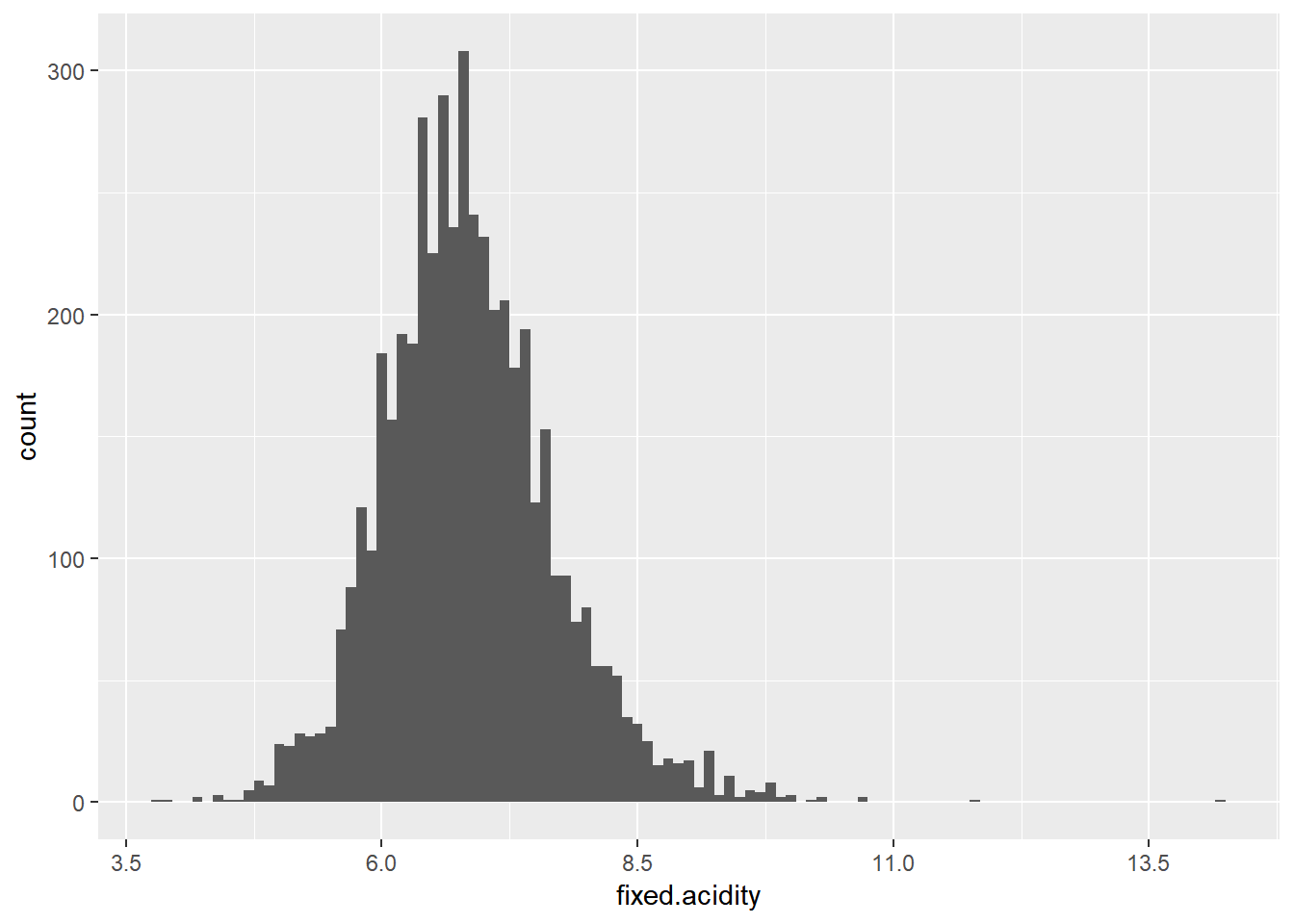## 挥发性酸（volatile acidity）

``````## 绘制挥发性酸含量分布图
ggplot(aes(x = volatile.acidity), data = df) +
geom_histogram(binwidth = 0.01) +
scale_x_continuous(breaks = seq(0, 1.2, 0.2))
``````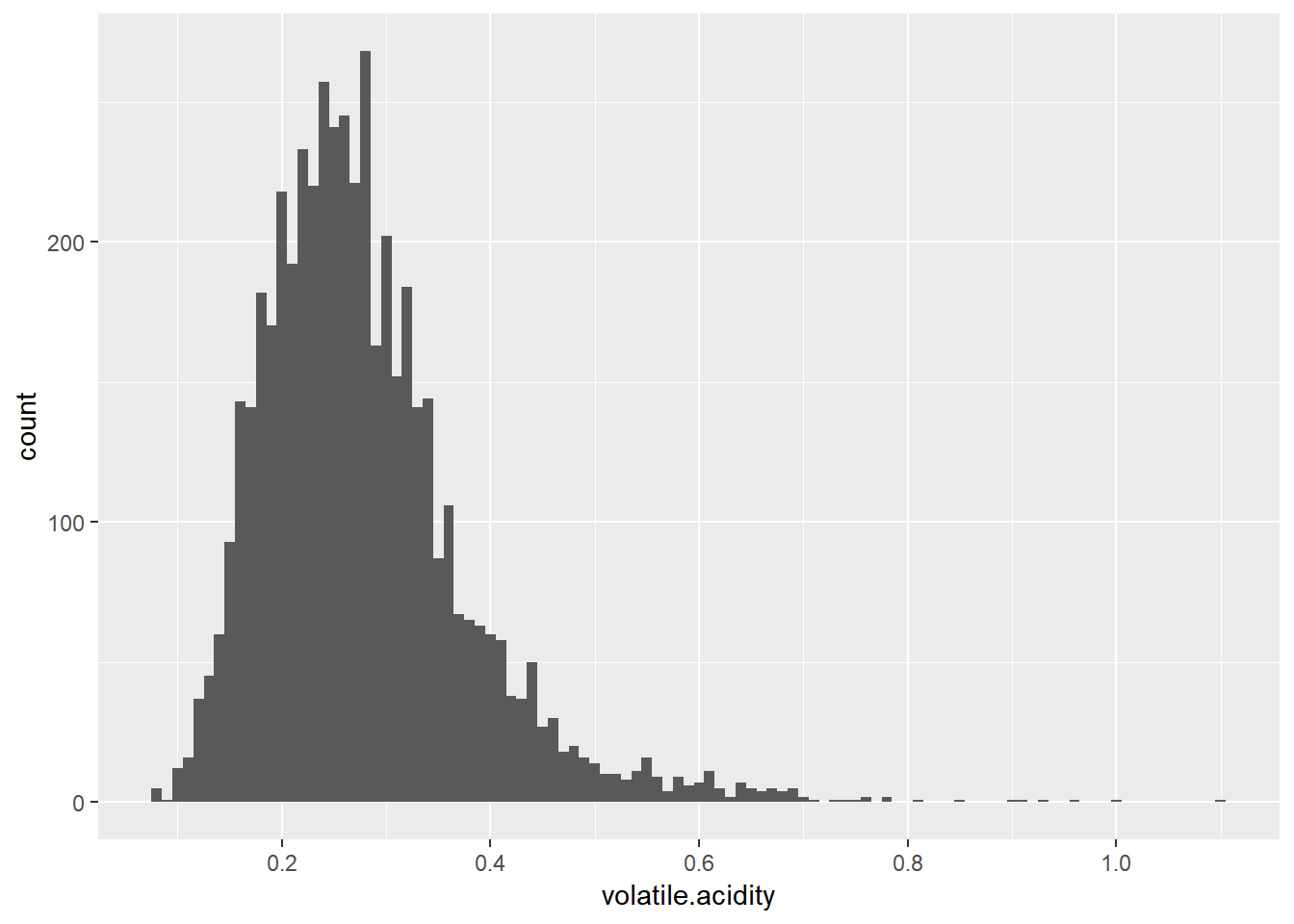## 柠檬酸（citric acid）

``````## 绘制柠檬酸含量分布图
ggplot(aes(x = citric.acid), data = df) +
geom_histogram(binwidth = 0.01) +
scale_x_continuous(breaks = seq(0, 1.8, 0.2))
``````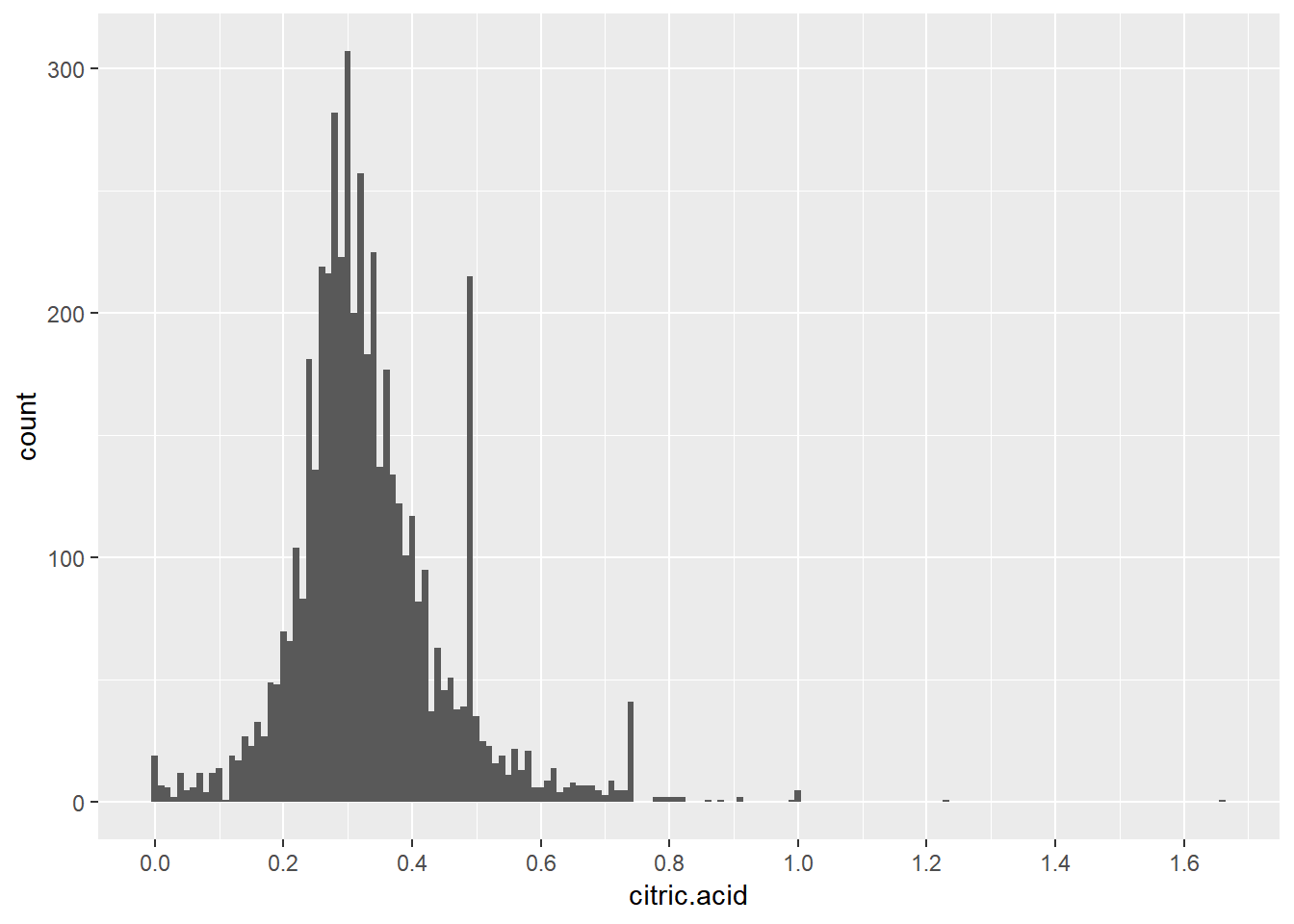## 残余糖分（residual sugar）

``````## 绘制残余糖分含量分布图
ggplot(aes(x = residual.sugar), data = df) +
geom_histogram(binwidth = 0.5) +
scale_x_continuous(breaks = seq(0, 66, 5))
``````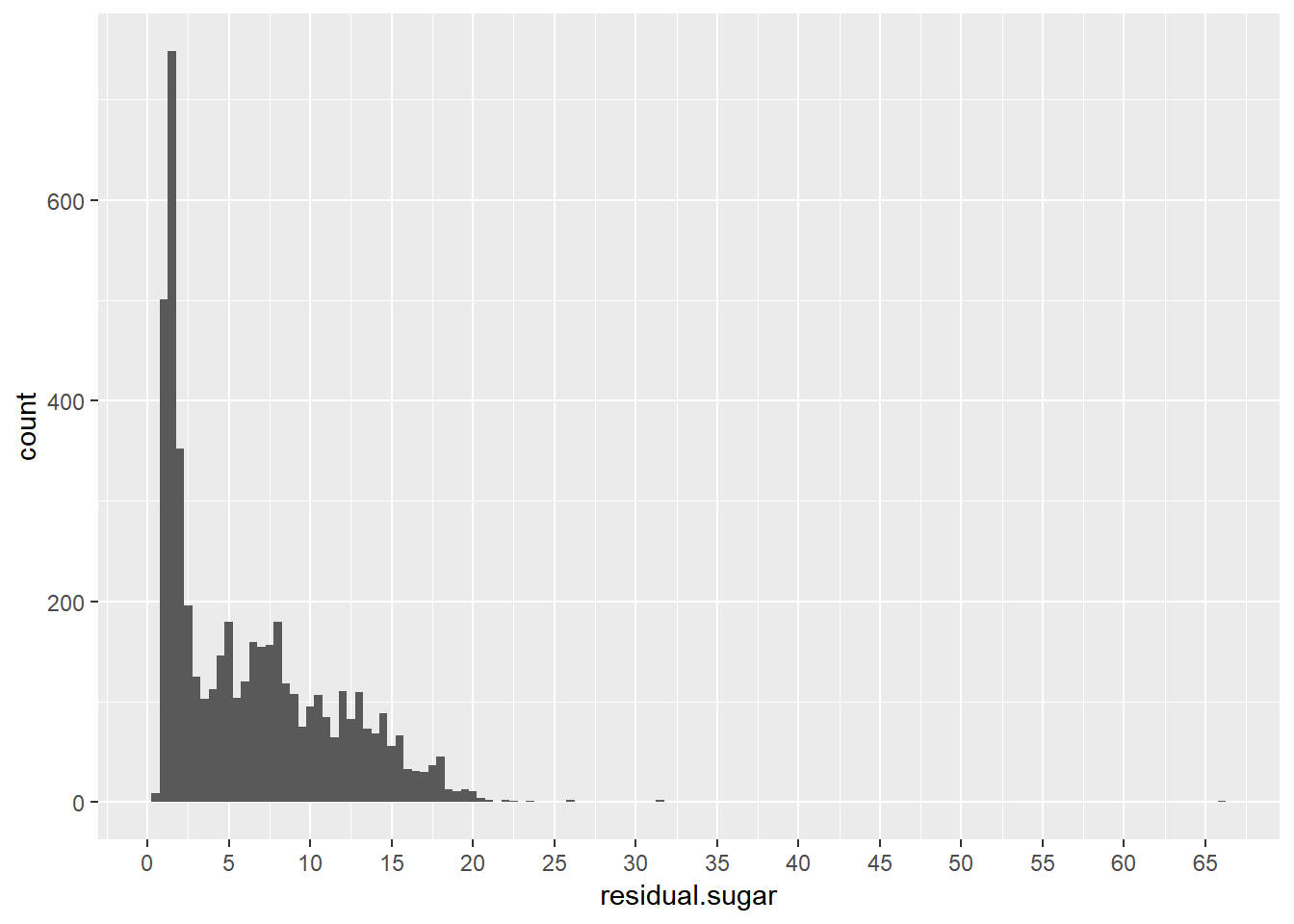``````## 绘制残余糖分含量分布图（对数坐标）
ggplot(aes(x = residual.sugar), data = df) +
geom_histogram(binwidth = 0.05) +
scale_x_log10() +
xlab(label = "residual sugar(log10)")
``````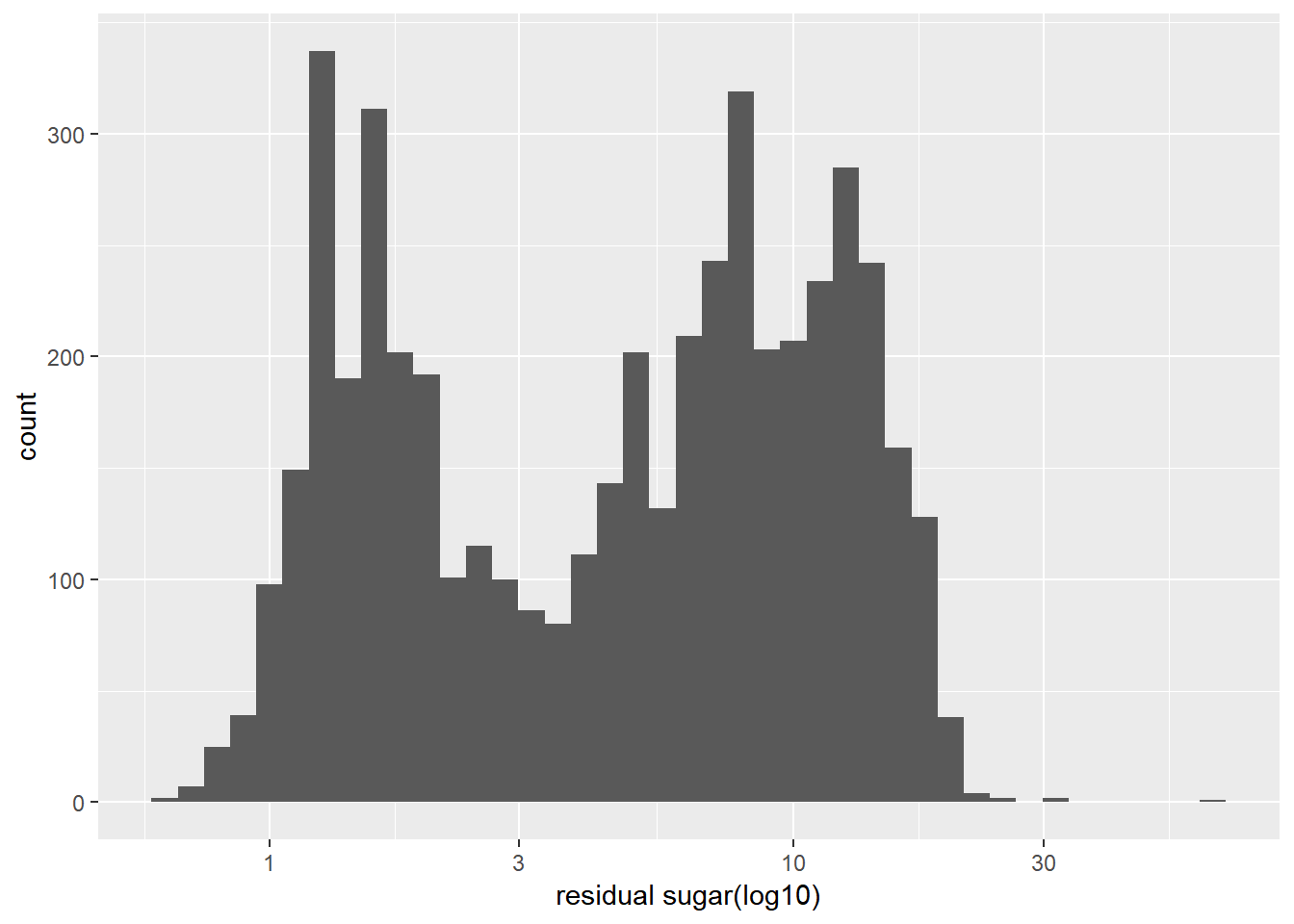``````#计算白葡萄酒的干型和甜型数据
df\$type<-ifelse(df\$residual.sugar >= 5.200, 'sweet', 'dry')

ggplot(aes(x = type), data = df) +
geom_bar(width = 0.3)

## 统计甜型和干型数量
table(df\$type)
``````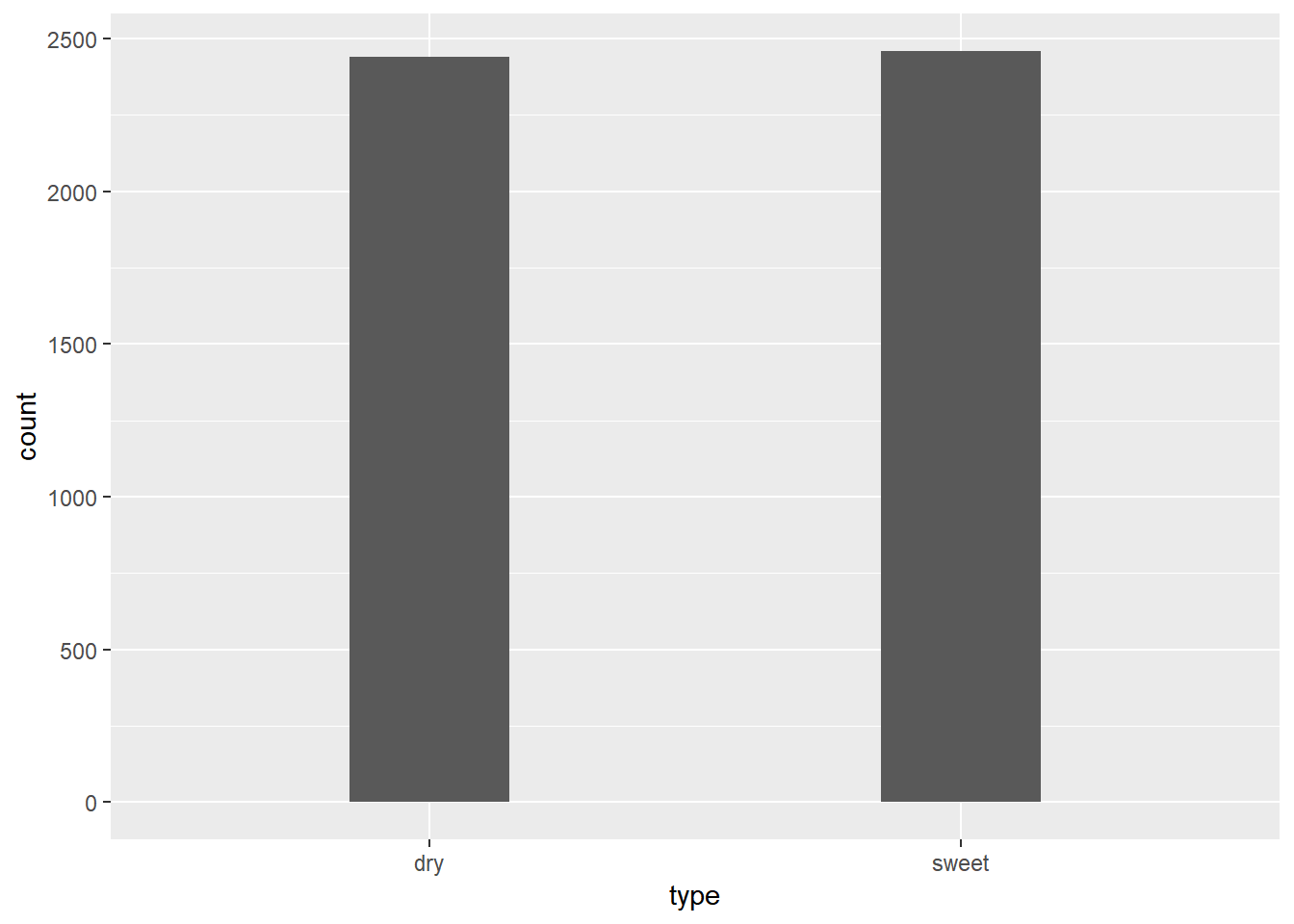``````##   dry sweet
##  2440  2458
``````

## 氯化物（chlorides）

``````## 绘制氯化物含量分布图
ggplot(aes(x = chlorides), data = df) +
geom_histogram(binwidth = 0.002) +
scale_x_continuous(breaks = seq(0, 0.4, 0.05))
``````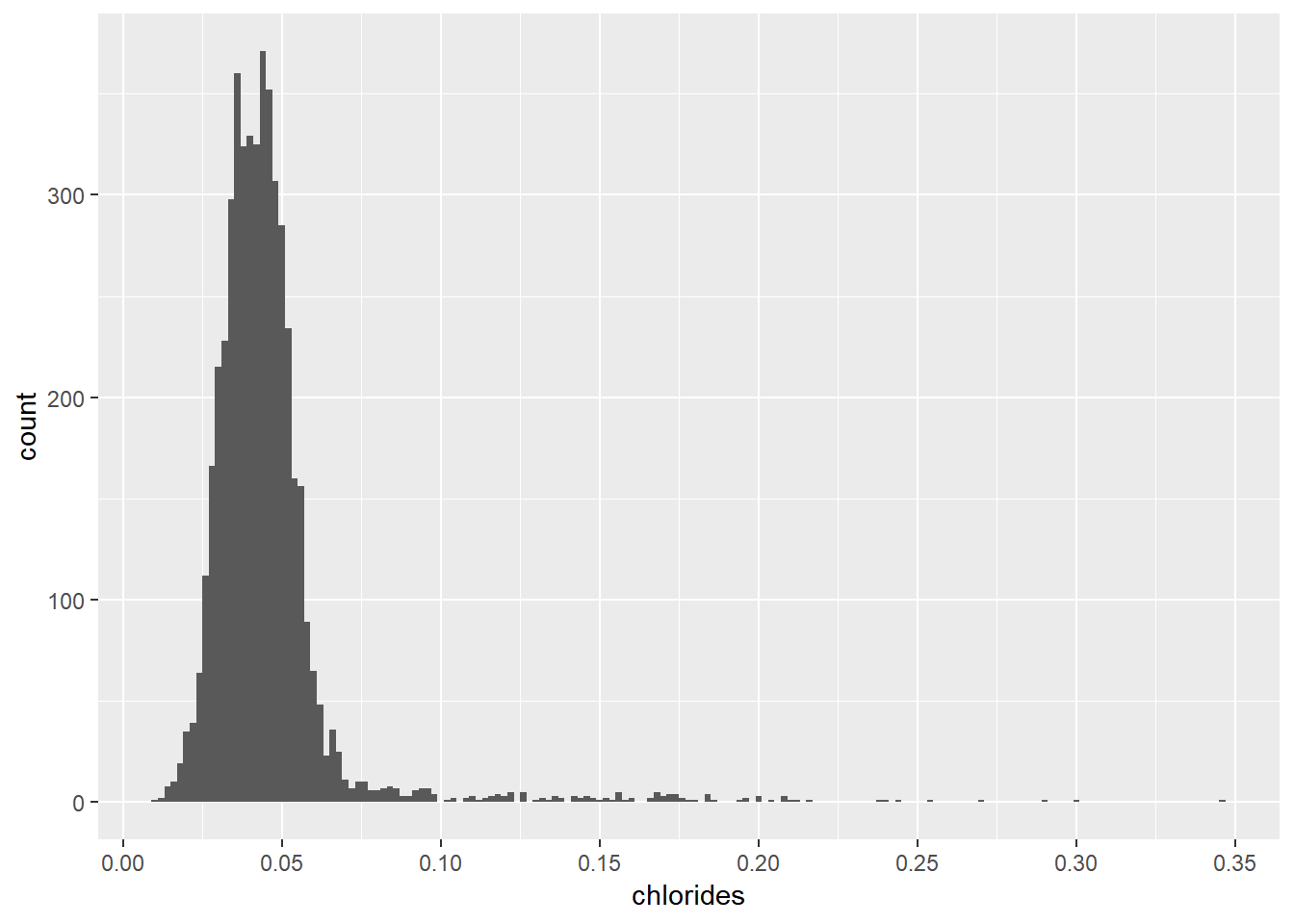## 游离二氧化硫（free sulfur dioxide）

``````## 绘制游离二氧化硫含量分布图
ggplot(aes(x = free.sulfur.dioxide), data = df) +
geom_histogram(binwidth = 2) +
scale_x_continuous(breaks = seq(0, 300, 30))
``````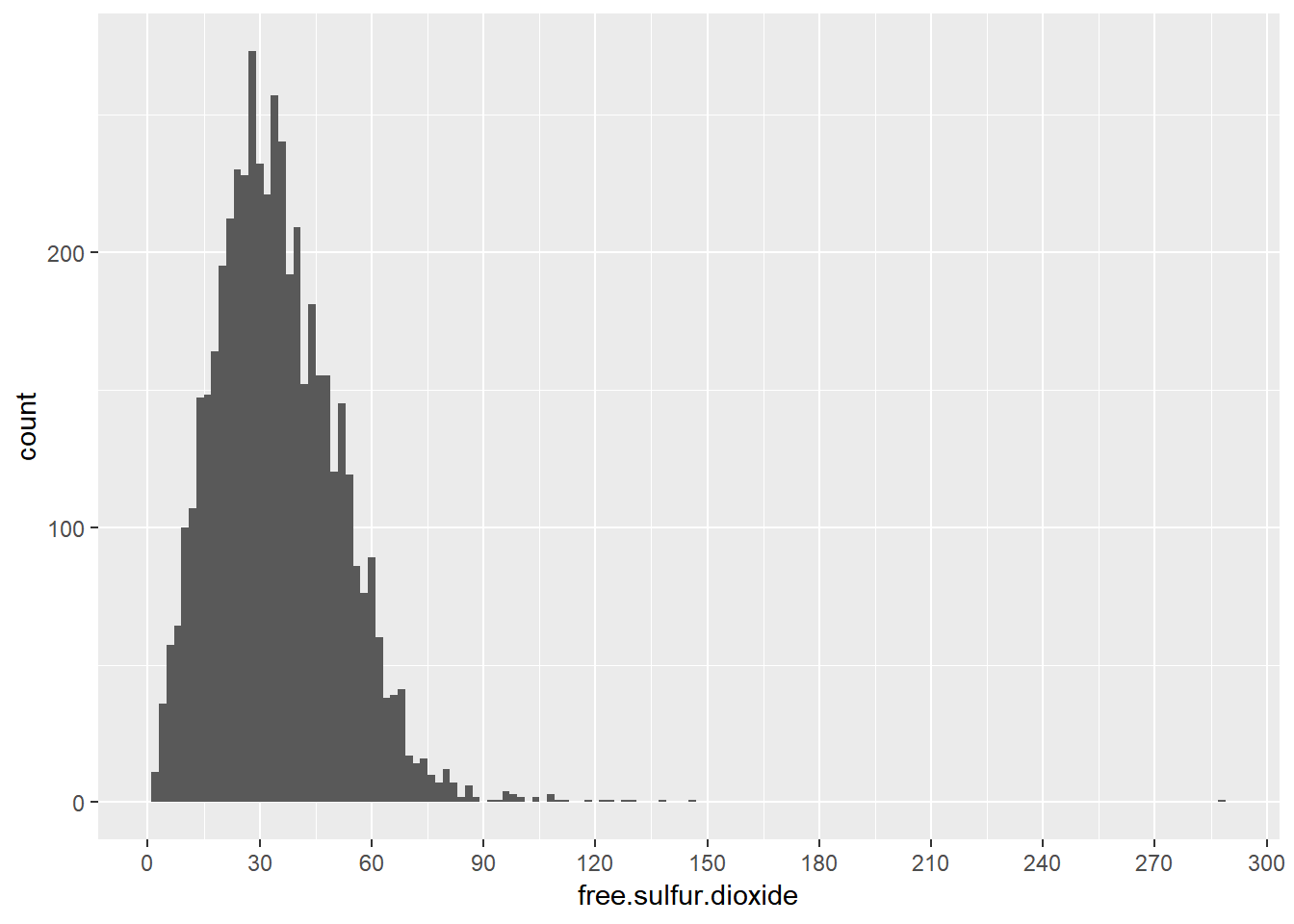## 总二氧化硫（total sulfur dioxide）

``````## 绘制总二氧化硫含量分布图
ggplot(aes(x = total.sulfur.dioxide), data = df) +
geom_histogram(binwidth = 5) +
scale_x_continuous(breaks = seq(0, 450, 40))
``````## 密度（density）

``````## 绘制密度分布图
ggplot(aes(x = density), data = df) +
geom_histogram(binwidth = 0.0005) +
scale_x_continuous(breaks = seq(0.9, 1.05, 0.005))
``````## pH

``````## 绘制pH值分布图
ggplot(aes(x = pH), data = df) +
geom_histogram(binwidth = 0.01) +
scale_x_continuous(breaks = seq(2.7, 3.9, 0.1))
``````

## 硫酸盐（sulphates）

``````## 绘制硫酸盐含量分布图
ggplot(aes(x = sulphates), data = df) +
geom_histogram(binwidth = 0.01) +
scale_x_continuous(breaks = seq(0.2, 1.1, 0.05))
``````

## 酒精度（alcohol）

``````## 绘制酒精度分布图
ggplot(aes(x = alcohol), data = df) +
geom_histogram(binwidth = 0.1) +
scale_x_continuous(breaks = seq(7, 15, 1))
``````

## 品质（quality）

``````## 绘制品质分布图
ggplot(aes(x = quality), data = df) +
geom_histogram(binwidth = 0.5) +
scale_x_continuous(breaks = seq(0, 10, 1))
``````

``````## 给葡萄酒分出等级并绘制统计图
df\$rating <- ifelse(df\$quality >= 8, 'good', ifelse(df\$quality == 7, 'above-average',ifelse(df\$quality == 6,"average",ifelse(df\$quality == 5,"below-average","bad"))))

ggplot(aes(x = rating), data = df) +
geom_bar(width = 0.3)

## 统计数据
table(df\$rating)
``````

``````## 选取干型白葡萄酒
dry=filter(df,type == "dry")
table(dry\$rating)

## 选取甜型葡萄酒
sweet=filter(df,type == "sweet")
table(sweet\$rating)
``````

## 双变量分析

``````## 画出变量之间的相关系数图
ggcorr(data = subset(df, select = -c(X,type,rating)), # 去除不需要的特征变量后再做图
label = TRUE,
label_size = 4,
label_round = 2,
label_alpha = TRUE,
hjust = 0.8,
size = 3)
``````

ph值和固定酸含量的相关性较大，却与其他酸含量关系不大。

## 残余糖分与密度

``````## 画出残余糖分与密度的关系（散点图）
ggplot(aes(x = residual.sugar, y = density), data = df) +
geom_point(alpha = 0.1) +
geom_smooth(method = 'lm', color = 'blue') + # 添加回归曲线
xlim(min(df\$residual.sugar), quantile(df\$residual.sugar, 0.95)) +
ylim(min(df\$density), quantile(df\$density, 0.95))

cor(df\$residual.sugar, df\$density)
``````

``````## 画出残余糖分与密度的关系（散点图）干型白葡萄酒
ggplot(aes(x = residual.sugar, y = density), data = dry) +
geom_point(alpha = 0.1) +
geom_smooth(method = 'lm', color = 'blue') + # 添加回归曲线
xlim(min(dry\$residual.sugar), quantile(dry\$residual.sugar, 0.95)) +
ylim(min(dry\$density), quantile(dry\$density, 0.95))

cor(dry\$residual.sugar, dry\$density)
``````

``````## 画出残余糖分与密度的关系（散点图）甜型白葡萄酒
ggplot(aes(x = residual.sugar, y = density), data = sweet) +
geom_point(alpha = 0.1) +
geom_smooth(method = 'lm', color = 'blue') +
xlim(min(sweet\$residual.sugar), quantile(sweet\$residual.sugar, 0.95)) +
ylim(min(sweet\$density), quantile(sweet\$density, 0.95))

cor(sweet\$residual.sugar, sweet\$density)
``````

## 残余糖分与酒精度

``````## 画出残余糖分与酒精度的关系（散点图）
ggplot(aes(x = residual.sugar, y = alcohol), data = df) +
geom_point(alpha = 0.1) +
geom_smooth(method = 'lm', color = 'blue') + # 添加回归曲线
xlim(min(df\$residual.sugar), quantile(df\$residual.sugar, 0.95)) +
ylim(min(df\$alcohol), quantile(df\$alcohol, 0.95))

cor(df\$residual.sugar, df\$alcohol)
``````

## 密度与酒精度

``````## 画出密度与酒精度的关系（散点图）
ggplot(aes(x = density, y = alcohol), data = df) +
geom_point(alpha = 0.1) +
geom_smooth(method = 'lm', color = 'blue') + # 添加回归曲线
xlim(min(df\$density), quantile(df\$density, 0.95)) +
ylim(min(df\$alcohol), quantile(df\$alcohol, 0.95))

cor(df\$density, df\$alcohol)
``````

## 酒精度与总二氧化硫

``````## 画出总二氧化硫度与酒精度的关系（散点图）
ggplot(aes(x = total.sulfur.dioxide, y = alcohol), data = df) +
geom_point(alpha = 0.1) +
geom_smooth(method = 'lm', color = 'blue') + # 添加回归曲线
xlim(min(df\$total.sulfur.dioxide), quantile(df\$total.sulfur.dioxide, 0.95)) +
ylim(min(df\$alcohol), quantile(df\$alcohol, 0.95))

cor(df\$total.sulfur.dioxide, df\$alcohol)
``````

## 游离二氧化硫和总二氧化硫

``````## 画出游离二氧化硫和总二氧化硫的关系（散点图）
ggplot(aes(x = free.sulfur.dioxide, y = total.sulfur.dioxide), data = df) +
geom_point(alpha = 0.1) +
geom_smooth(method = 'lm', color = 'blue') + # 添加回归曲线
xlim(min(df\$free.sulfur.dioxide), quantile(df\$free.sulfur.dioxide, 0.95)) +
ylim(min(df\$total.sulfur.dioxide), quantile(df\$total.sulfur.dioxide, 0.95))

cor(df\$free.sulfur.dioxide, df\$total.sulfur.dioxide)
``````

## pH值与固定酸

``````## 画出pH值与固定酸的关系（散点图）
ggplot(aes(x = fixed.acidity, y = pH), data = df) +
geom_point(alpha = 0.1) +
geom_smooth(method = 'lm', color = 'blue') + # 添加回归曲线
xlim(min(df\$fixed.acidity), quantile(df\$fixed.acidity, 0.95)) +
ylim(min(df\$pH), quantile(df\$pH, 0.95))

cor(df\$fixed.acidity, df\$pH)
``````

## 品质与酒精度

``````## 添加quality.factor
df\$quality.factor <- factor(df\$quality)

# 画出品质与酒精度箱形图
ggplot(aes(x = quality.factor, y = alcohol), data = df) +
geom_boxplot() +
xlab('quality')
``````

## 品质与密度

``````# 画出品质与密度含量箱形图
ggplot(aes(x = quality.factor, y = density), data = df) +
geom_boxplot() +
ylim(0.99,1.001) +
xlab('quality')
``````

## 品质与氯化物

``````## 画出品质与氯化物含量箱形图
ggplot(aes(x = quality.factor, y = chlorides), data = df) +
geom_boxplot() +
ylim(min(df\$chlorides), quantile(df\$chlorides, 0.95)) +
xlab('quality')
``````

## 品质与干型和甜型的关系

``````# 画出葡萄酒类型（干，甜）与品质关系的分布图
ggplot(aes(fill = type, x = quality), data = df)+
geom_bar(position = "dodge")
``````

# 多变量绘图选择

## 酒精度，品质和残余糖分

``````# 画出酒精，品质，酒的类型（干，甜）之间的箱形图
ggplot(aes(x = quality.factor, y = alcohol), data = df) +
geom_boxplot(aes(color = df\$type)) +
xlab('quality')

``````

## 酒精度、残余糖分与密度

``````## 绘制酒精度、残余糖分与密度三元图
ggtern(aes(x = df\$residual.sugar,y = df\$alcohol,z = df\$density),data = df,shape = group,colour = group) +
theme_rgbw() +
geom_point(alpha = 0.1) +
theme_legend_position("topright") +
xlab("residual.sugar") + ylab("alcohol") + zlab("density")

``````

## 酒精度、氯化物与品质

``````## 绘制酒精度、氯化物与品质 三元图
ggtern(aes(x = df\$chlorides,y = df\$alcohol,z = df\$quality),data = df,shape = group,colour = group) +
theme_rgbw() +
geom_point(alpha = 0.1) +
theme_legend_position("topright") +
xlab("chlorides") + ylab("alcohol") + zlab("quality")

``````

# 定稿图与总结

### 绘图一

``````## 画出变量之间的相关系数图
ggcorr(data = subset(df, select = -c(X,type,rating)), # 去除不需要的特征变量后再做图
label = TRUE,
label_size = 4,
label_round = 2,
label_alpha = TRUE,
hjust = 0.8,
size = 3) +
labs(title ='Relationship among all data')
``````

### 绘图二

``````## 画出酒精，品质，酒的类型（干，甜）之间的箱形图
ggplot(aes(x = quality.factor, y = alcohol), data = df) +
geom_boxplot(aes(color = df\$type)) +
labs(x = 'Quality',
y = 'Alcohol (% by volume)',
title ='Relationship of Quality and Alcohol')
``````

### 绘图三

``````# 画出葡萄酒类型（干，甜）与品质关系的分布图
ggplot(aes(fill = type, x = quality), data = df)+
geom_bar(position = "dodge") +
labs(x = 'Quality',
y = 'Type Count',
title ='Quality Counts of Dry and Sweet')

``````

# 参考资料

1. 揭秘葡萄酒中的糖分

2.使用dplyr进行数据操作（30个实例）